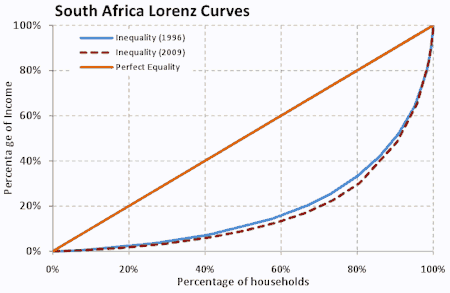Every time I talk about the Gini coefficient, I get vaguely excited. Sure, I’m always disappointed by the “coefficient” part of that phrase – but “Gini” sounds like a neologistic contraction of “gin” and “mini”; being an apt yet euphemistic description for the amount of Bombay Sapphire in my lunchtime tonic.

And if you assumed that the Gini coefficient was some strange statistical measure for “who gets to drink the G&Ts at lunchtime?”, then you actually wouldn’t be that far from the truth… Because the Gini is actually the generally-accepted measure of income inequality.

And if you’re wondering how it is that you can get a single number to be a clear indicator of income distribution (“surely you need to look at a graph?”), then read on.

If The Measure Were Going To Be A Single Number…

So let’s just assume that we wanted a way to compare the inequalities between countries at a glance, because countries that have too much income condensed in the top 20% of the population sound a lot like:

• France, immediately prior to the French Revolution;
• Russia, immediately prior to the Russian Revolution;
• Argentina, immediately prior to its military junta takeover;
• Any African country that was about to undergo a liberation struggle.

So measuring the income dispersion in a population would give us a pretty strong indicator of how soon we could expect an uprising.

It’s difficult to rank graphs, so we’d have to condense this:Into a single number.

And you might pause for breath, and say “well maybe we should just look at how much wealth is held by the highest fifth of the population?”

Which sounds reasonable – only, if the rest of the wealth is split quite evenly between the balance of the 80%, that might get a different political response to the rest of the wealth being held the second-highest fifth…

So we’d actually like to get an idea of how much income is held across the board.

Also, we’d probably need a scale. Of, say, 0 to 1 (or 100%), where:

• A Gini Coefficient of “0” would mean that income is equally split between the entire population (ie. perfect equality); and
• A Gini Coefficient of “1” would mean that all the income belongs to one person, and everyone else is his/her serf (ie. perfect inequality).

The Solution

Involves a Lorenz Curve and gradients.

I know – just bear with me.

So someone clever (sounds to me like his name was Lorenz) said something like: “seems to me what we need is a way of showing how all the income is distributed”. And he focused on the word “all”, and drew a graph that measured income cumulatively rather than discretely. It looked like this:What it’s saying:

• On the bottom of the graph (the x axis), you have the entire population, arranged in order from poorest to richest.
• On the side of the graph (the y axis), you have all the income.
• Each point on the graph will measure how much of the income is held by that percentage of the population (ie. the middle point will show how much of the income is held by the bottom 50% of the population; the point at the end will show that 100% of the population holds 100% of the income – because, like, duh).

What we can say about the graph:

• A straight line would tell you that the income is split evenly (each additional percentage point of people adds an equal percentage point of income).
• The more curved the line, the less income is held by the lower classes, and (therefore) the more income is held by the higher classes.

So here’s the smart part: you start off with the assumption of perfect equality (zero), and then measure how much the curve deviates from the line to get a measure of how bad the income inequality is. Let’s call the area of deviation “A”, and the area of perfect equality “A + B”. And the ratio would be:

A
A + BThe smaller A is, the closer the ratio will be to 0 (perfect equality). The larger A is, the closer the ration will be to 1 (perfect inequality).

Given that piece of information, here’s a graph that I stole from Wikipedia, showing Gini coefficients the world over:How’s that for an indicator of social unrest?